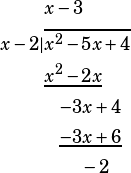From the new blue book, test #1, section 4, #29:
For a polynomial p(x), the value of p(3) is −2. Which of the following must be true about p(x) ?
A) x − 5 is a factor of p(x).
B) x − 2 is a factor of p(x).
C) x + 2 is a factor of p(x).
D) The remainder when p(x) is divided by x − 3 is −2.

Yeah, this is a tricky one. I actually think the way many students will get it is by elimination. Given p(3) = –2, we know nothing about x – 5, x – 2, or x + 2. So, choice D might not be intuitive, but at least it’s related to p(3) somehow.

Remember that if p(3) = 0, then we know that x – 3 is a factor of p(x). Here’s the tricky bit: if p(3) = –2, then p(3) + 2 = 0. In other words, if we shift p(x) up 2, then we’ll have a polynomial that’s divisible by x – 3. If that’s the case, then the remainder when p(x) is divided by x – 3 must be –2.

Really, the best way to understand this is to see an example. Let’s make up a function:. We can FOIL that out:. When we graph that, we see that there are zeros at x = 2 and x = 3. And because we made the function the way we did, we already know that it’s divisible by x – 2 and x – 3.Now, what happens when we shift that function down by 2?. Let’s call that g(x). Obviously now we don’t have zeros at x = 2 and x = 3. We have –2s instead: g(2) = g(3) = –2.What happens when we divide g(x) by x – 2?That’s right—we get x – 3 with a remainder of –2.zevms1 says:

That’s an interesting way to approach the problem. Students could also just know/review/memorize the Polynomial Remainder Theorem, which is usually taught in Alg 2 or pre-calc. When you evaluate a function at a given value n, the result is the remainder when you divide the function by the linear factor x-n corresponding to that value. In other words, if f(n) = c, then c is the remainder when f is divided by x-n. In the example above, p(3) = -2, so -2 is the remainder when p is divided by x-3. This would also help if ETS asked a question such as, “what is the remainder when f(x) = x^2 + 3x – 8 is divided by x-3?” Instead of doing long or synthetic division, just evaluate f(3) et voila, you have your remainder of 10. It’s a bit tricky perhaps to grasp at first, but with a bit of practice shouldn’t be too tough to master. Again the solution above is clever, but I think many students would be hard-pressed to work through that kind of solution in the heat of the moment on test day.Mike McClenathan says:

Thanks for this comment. I should have made it more clear that what I was trying to do in the post above is arrive at that theorem, which I agree is useful for this and similar polynomial questions.Hmmm says:

So, just to make sure I understand this concept, would you tell me if I am thinking about this correctly? if I were trying to do this problem as efficiently as possible, I would look at the answer choices after reading the question. Then, my thinking process would be something like this:

A) “x − 5 is a factor of p(x).” If this must be true for p(x), then p(5) = 0. However, I have no way of knowing this since all I know about the p function is that p(3) = -2

B) “x − 2 is a factor of p(x).” Similar to A. If this were true, then p(2) = 0, but I have no way of knowing whether this must be true from information given.

C) “x + 2 is a factor of p(x).” Again, if this were true, p(-2) =0, but I have no way of knowing this.

D) “The remainder when p(x) is divided by x − 3 is −2.” If this is true, then p(3) = -2, which is true because it was given in the question.

Is that right? This certainly seems harder than the old SAT, but I guess it makes sense. Hopefully, it’s just a random weird problem.Mike McClenathan says:

I’d say that’s a perfect thought process!